# Impact of Newtonian Heating on MHD Micropolar Fluid for the Influence of Heat Source

K.K. Prusty1, S.N. Sahoo1, S.R. Mishra1, *
1 Department of Mathematics, Siksha ‘O’ Anusandhan Deemed to be University, Bhubaneswar-751030, Odisha, India

#### Article Metrics

0
##### Total Statistics:

Full-Text HTML Views: 567
Abstract HTML Views: 208
##### Unique Statistics:

Full-Text HTML Views: 283
Abstract HTML Views: 162open-access license: This is an open access article distributed under the terms of the Creative Commons Attribution 4.0 International Public License (CC-BY 4.0), a copy of which is available at: https://creativecommons.org/licenses/by/4.0/legalcode. This license permits unrestricted use, distribution, and reproduction in any medium, provided the original author and source are credited.

* Address correspondence to this author at the Department of Mathematics, Siksha ‘O’ Anusandhan Deemed to be University, Bhubaneswar-751030, Odisha, India; E-mail: satyaranjan_mshr@yahoo.co.in

## Abstract

### Introduction:

The current analysis deals with the flow of time-independent conducting micropolar fluid past a stretching sheet. Furthermore, the influences of magnetic field strength and heat sources are also discussed in this study. The current study is important for the growing applications of magnetism in various chemical systems like pumping, transportation, trapping, sorting, separation, etc.

### Aims:

In addition, the objective of the present exploration is the consideration of Newtonian heating conditions.

### Methods:

Transformation of nonlinear PDEs of the flow phenomena into nonlinear coupled ODEs is done using suitable similarity variables, and these are handled by employing the “Runge-Kutta fourth-order” scheme in association with the “shooting technique”. The influence of flow characteristics proposed in this study is analyzed via graphs. The engineering coefficients for various parameters are simulated numerically and deployed through the table.

### Results:

Finally, it was found that the rotational viscosity dominates over the fluid viscosity and enhances the momentum profile.

### Conclusion:

An augmentation in the Prandtl number retards the fluid temperature and the application of applied magnetic field built up the resistance that retards fluid momentum.

Keywords: Micropolar fluid, MHD flow, Newtonian heating, Heat source, Numerical treatment, Microrotation.

## 1. INTRODUCTION

The recent era of science advances the emerging research areas based upon the application of polar fluids and dynamics relating to them. The specialty of this liquid is that several molecules are randomly oriented in the mixture of a fluid for which every part of the fluid molecule has its rotation and translation. Upon analysis, several interesting facts are developed that cannot be studied just by considering the earlier Newtonian fluids. For the appropriate suspensions, the models of micropolar fluids have an important role, and such fluids are biological fluids like blood flow, animal blood, etc., and lubricating oils. These particles experience a few micro rotations, such as rotational inertia, where various aspects of stress are vital for polar liquids. Depending upon the conservation laws for the constitutive equations, the impact of microrotation, as well as rotational inertia, is important. Therefore, both the momentum vectors, i.e., axial and angular momentum, are combined in the governing equation. For the prediction of micro-scale characteristics, the application of these micropolar fluids is vital and using a microrotation vector, the rotations can be further explained.

Due to the several applications in various areas on the flow of micropolar fluid over an expanding surface over the last decade, several researchers have devoted their contributions. Several examples can be considered for the applications, such as the manufacture of glass sheets, materials applied in metallurgy used for thermal and moisture treatment, and textile usages for processing polymers. For polymer processing, several research works have been conducted on the polar fluid by considering various aspects of chemical industries. Furthermore, the use of micropolar fluid has a crucial role in polymer extrusion, cable coating, and similar other processes in which heat transfer occurs through the stretching surface. As an experimental investigation, Crane  investigated the polar fluid over an expanding sheet. Based on his study, several authors have extended the study in several directions imposing different physical properties to eliminate the heat transport phenomenon. For every industrial process, the production process deals with the rate of heat transfer. Several studies [2-8] have been conducted on the flow of polar fluid. Free convection of polar fluid considering the Newtonian heating boundary condition over a vertical surface is studied by Chaudhary and Jain . Makinde  examined the magnetohydrodynamic time-dependent flow for the various aspects of heat transfer properties using Newtonian heating under Navier slip conditions. An experimental investigation was conducted by Salleh et al. in a heat transfer on the boundary layer flow . They have employed the Keller box numerical approach to solve the system of nonlinear governing equations. The stagnation point flow of polar fluid over a stretching surface is investigated by Nazar et al. . Furthermore, Ishak et al.  extended their work on the time-independent MHD free and forced convection over a vertical stretching surface by considering a polar fluid. Mishra et al.  carried out their analysis on the free convection of micropolar fluid over a shrinking surface to examine the impact of the heat source. They conducted their work numerically to determine the significant contribution of the parameters involved in the flow problem. Ishak et al.  also proposed the MHD flow of polar fluids. They investigated the constant wall heat flux where the flow past a wedge. Srinivasacharya and RamReddy  worked on the free convection of the flow and heat transfer of micropolar fluid that was doubly stratified. Recently, Shamshuddin et al.  investigated the flow of chemically-reacting micropolar fluid past an inclined permeable surface for the behavior of radiative heat. They employed “Lie symmetry analysis” and numerical solutions for solving complex nonlinear problems characterized by the governing equations.

However, referring to the aforementioned literature, it can be concluded that heat transfer on MHD micropolar liquid in the presence of Newtonian heating over an expanding sheet due to the inclusion of a heat source is reported rarely. Earlier, studies on the thermal efficiency of polar liquid by imposing Newtonian heating past an expanding sheet have been carried out, but the use of applied magnetic field and additional heat source effects are not considered. Therefore, recent analysis confined to the time-independent MHD micropolar fluid is considered for the interaction of additional heat source, and Newtonian heating boundary condition is also imposed.

## 2. MATERIALS AND METHODS

### 2.1. Formulation and Solution

A time-independent flow of micropolar fluid over an expanding sheet is analyzed in the present literature. It is assumed that the sheet is along the x -axis in the flow direction, and y-axis is transverse to it. Furthermore, sheet velocity is assumed to be uw(x) = ax, with stretching constant a, a real number. The constant magnetic strength B0 is imposed normally to the flow direction. The heat transport phenomenon is enhanced because of the consideration according to Newtonian heating conditions (Fig. 1).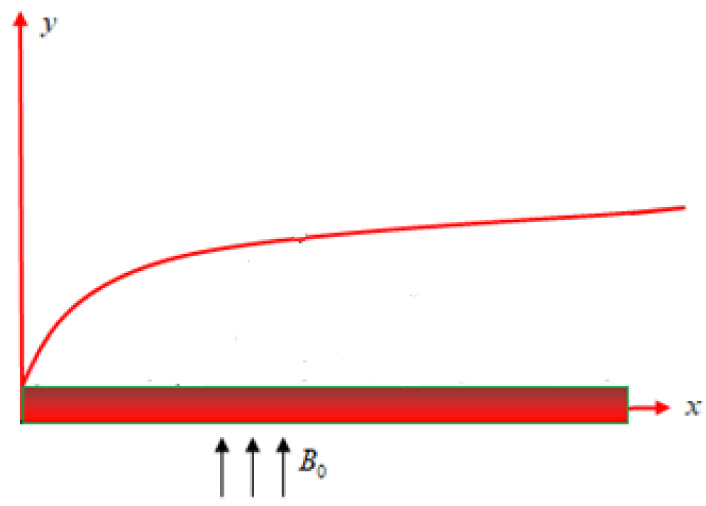Fig. 1. Flow geometry.

Following aforesaid assumptions, the constitutive flow equations associated with their boundary conditions are: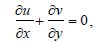(1)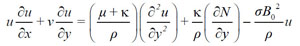(2)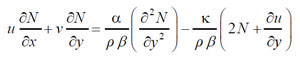(3)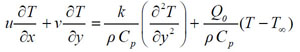(4)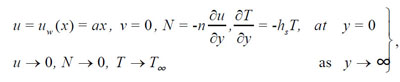(5)

where β=υ/aand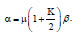, the non-Newtonian characteristic eliminates, and the fluid is viscous. Here, n, the rotational parameter and 0n1. For n = 0, the rotation for the microelements ceases and denotes their Fragile solutals at the surface of the sheet. However, the skew-symmetric stress tensor becomes extinct for n = 1/2, which points out the delicate microelement concentration. Finally, the laminarity is distressed for n = 1.

The similarity variable η is defined as: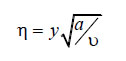(6)

The non-dimensional variables are defined as: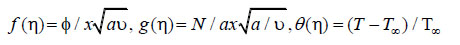(7)

The aforesaid transformations satisfy equation (1) automatically by consideringϕ(x,y)the stream function, i.e.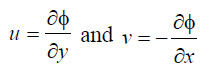(8)

Now, employing equations (6-8) and the stream function, the leading equations (2-4) are condensed to nonlinear coupled ODEs in conjunction with boundary conditions and are deployed as: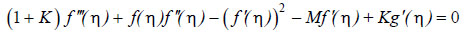(9)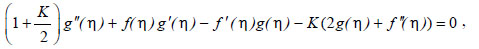(10)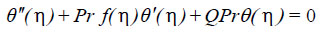(11)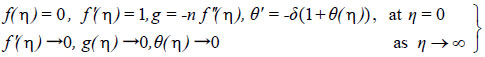(12)

where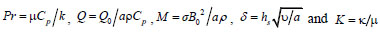.

The local shear stress coefficient is(13)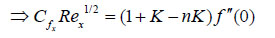(14)

The local couple stress (Mx)near the wall is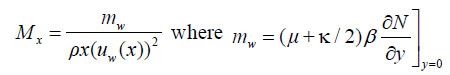(15)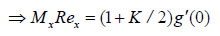(16)

Finally, the heat transfer rate (Nux) is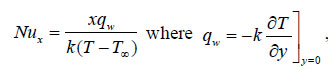(17)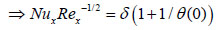(18)

where Rex=ax2/υ is the local Reynolds number.

## 3. RESULTS AND DISCUSSION

The present flow phenomena reveal the time-independent two-dimensional flow of conducting micropolar fluid to consider Newtonian heating conditions. Furthermore, the heat transport properties may be augmented with the inclusion of the heat source in the energy equation. The flow phenomena characterizing various profiles have been examined via graphs for the influence of a few of the imperative parameters involved in it. Also, the behaviors of the engineering coefficients are presented in the Table 1 illustrates the comparison of the current investigation with the work of Ramachandran et al.  in particular cases, and the result shows a good correlation.

Figs. (2 and 3) portray the influence of material parameter (K) on the axial velocity distribution for the wall condition parameter, n=0.5 and n=0 accordingly, as described in the earlier section. Here, the withdrawing material parameter, i.e., K =0, eliminates the non-Newtonian properties of the fluid. Therefore, from the figures, it is clarified that in the case of viscous fluid, the magnitude of the flow domain dominates over the non-Newtonian case. Moreover, higher values of the material parameter attenuate the profile significantly irrespective of the wall conditional parameter. Sudden fall within the flow domain is marked and occurs due to the dominating characteristic of the rotational viscosity over the fluid viscosity. The behavior of magnetization due to the several values of M on the fluid momentum and angular momentum is deployed via Figs. (4 and 5), respectively for n=0.5 and n=0. It indicates that the velocity profiles retard the insertion of the magnetic parameter. The fact is that the formation of resistive force sets in to reduce the profiles within the flow domain. The trend of the graphs is very similar. The impact of material parameters on angular momentum profiles is deliberated for n = 0.5, as presented in Fig. (6). It reveals that the angular velocity decelerates for 0<η<1.5 but increases for η>1.5 as the micropolar parameter increases. The mathematical expression for the material parameter suggests that for η>1.5, it varies as the vortex viscosity varies and becomes inverse with the fluid viscosity. Furthermore, for 0<η<1.5, the variation is opposite to that of the behavior presented due to the delicate concentration and vanishing environment for the microelements. Fig. (7) illustrates the variation of the angular velocity profile for the several values of the material parameter with n=0.0. A remarkable variation is exhibited for the nonexistence of the wall condition parameter. A hike in the angular momentum is exhibited near the surface of the sheet, and it further decreases gradually to reach the adequate boundary condition. The attenuation in the profile is shown for the augmentation in the material parameter. Fig. (8) depicts the behavior of heat energy distribution for various values of Pr. Noticeable retardation is marked in the fluid temperature because of the augmentation in Pr. Prandtl number is described as the relationship between the thermal and velocity boundary layer of the fluid. The amplification in Pr retards the thermal bounding surface thickness. The fact is the dominating behavior of the kinematic viscosity over the thermal diffusivity that resulted in the reduction in thickness. The structural presentation of the additional heat source/sink on the fluid temperature profile is exhibited in Figs. (9 and 10) respectively. Here, the positive sign, i.e., Q > 0, denotes the inclusion of heat generation and the negative, i.e., Q<0, depicts heat absorption. The interesting fact is that both the generation and absorption behave in opposite directions on the fluid temperature. A remarkable boost in the fluid temperature is exhibited for the heat source, whereas the retardation is marked for the heat sink. The characteristics of the Newtonian heating parameter (δ) on the energy profiles are exhibited in Fig. (11). A noticeable growth in the fluid temperature is marked due to the increase inδ. Moreover, the thermal boundary layer thickness decreases while increasingδ.

Table 1. Comparison of shear rate and the heat transfer rate for M= K=0.
f(0) θ(0)
Pr Ramachandran et al.  Present Ramachandran et al.  Present
0.71 1.7063 1.7062873 0.7641 0.7641176
7 1.5179 1.5179034 1.7224 1.7224724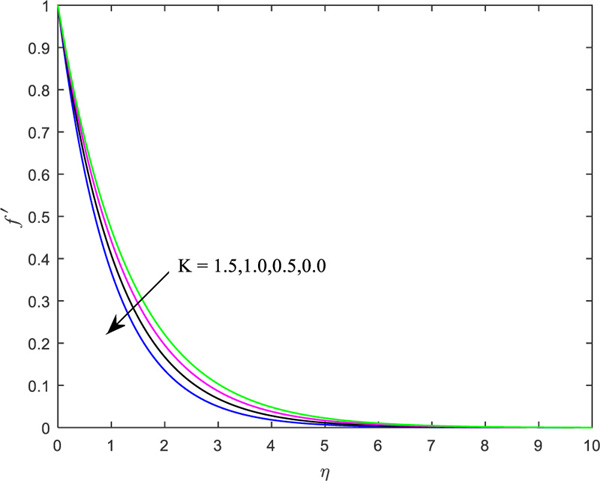Fig. (2). Influence of material parameter for n=0.5on the axial velocity.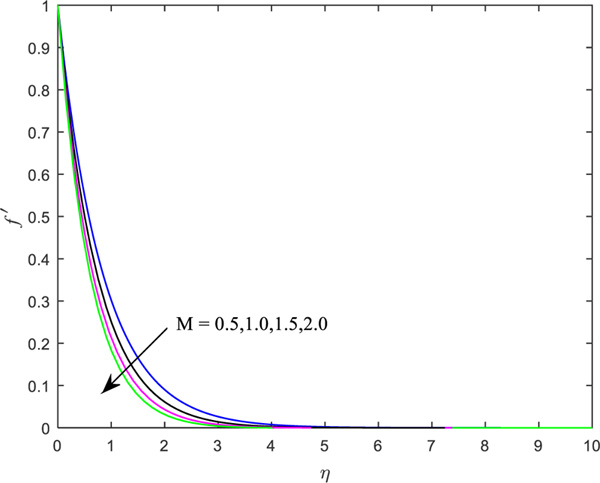Fig. (3). Influence of material parameter when n=0 on the axial velocity.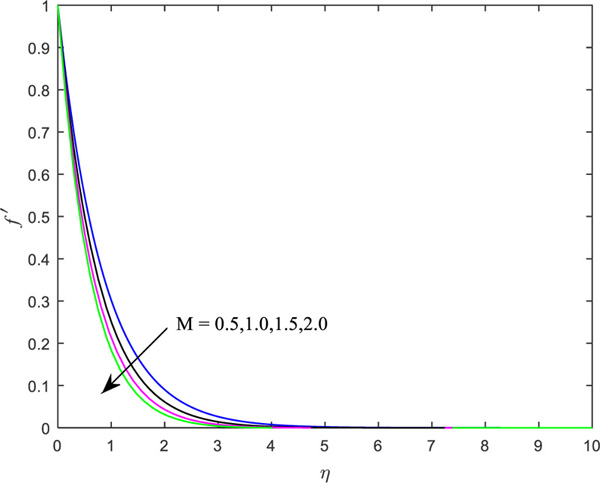Fig. (4). Influence of magnetic parameter for n=0.5 on the axial velocity.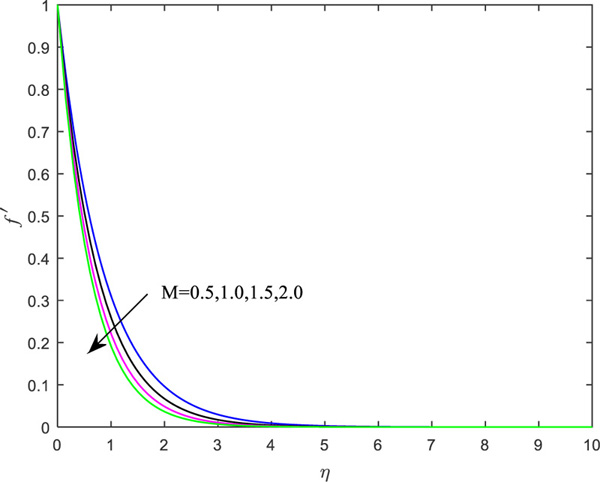Fig. (5). Behavior of magnetic parameter for n=0.0 on the axial velocity.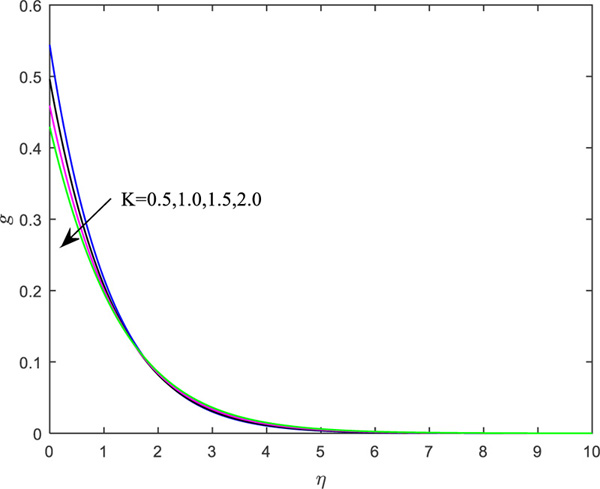Fig. (6). Influence of K on angular momentum for n=0.5.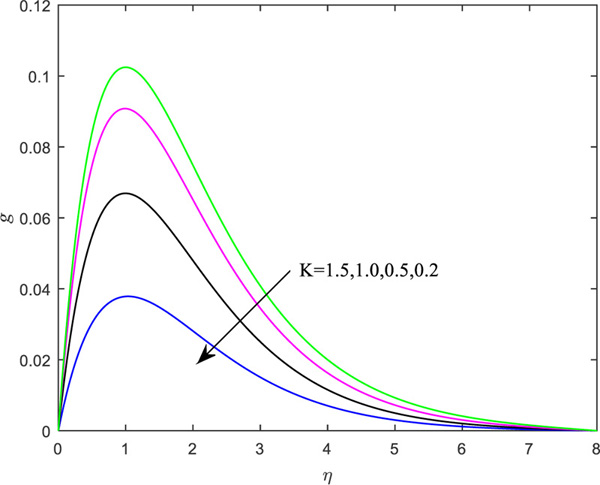Fig. (7). Influence of K on angular momentum for n=0.0.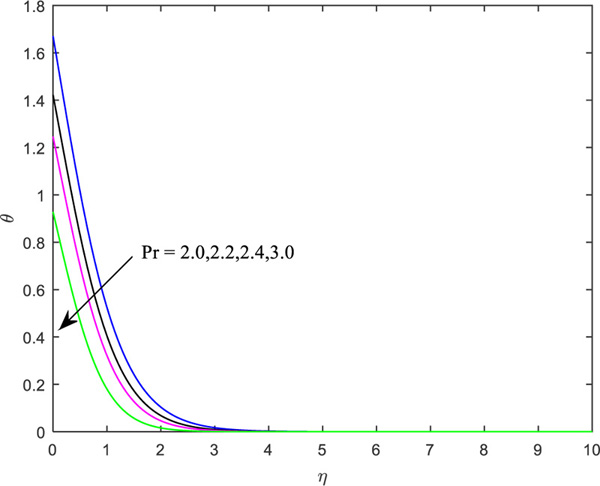Fig. (8). Temperature distributions for Pr.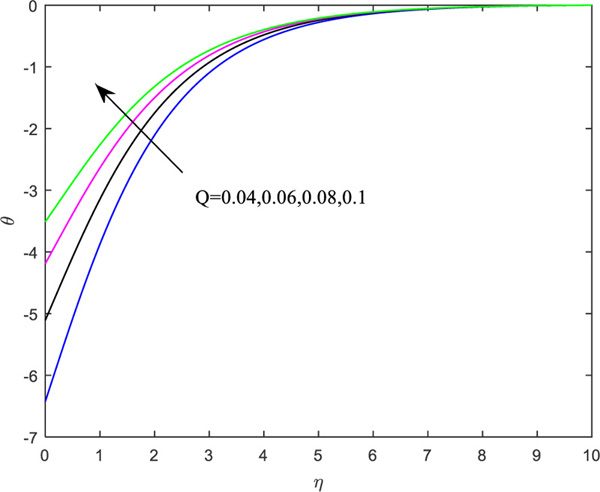Fig. (9). Behavior of heat source parameter.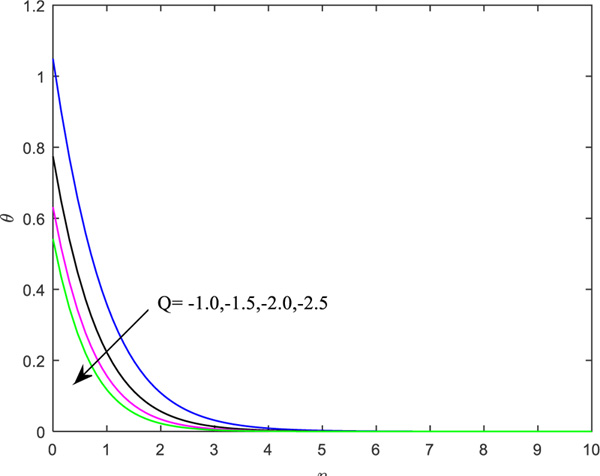Fig. (10). Behavior of heat sink.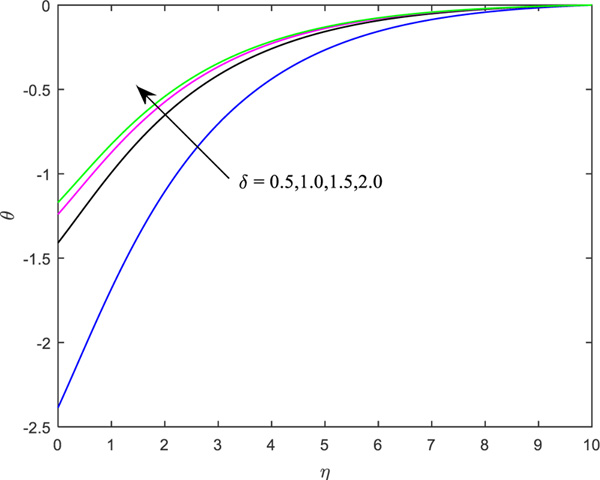Fig. (11). Effect of Newtonian heating parameter.

Table 2. Characteristics of engineering coefficients.
n K δ Pr Q M CfxRex1/2 Mx Rex Nux Rex-1/2
0.5 0.1 0.5 0.7 0.06 0.5 -1.25185 -0.59053 0.34896
0.7 -1.23887 -0.85783 0.34604
0.5 0.5 -1.36025 -0.61556 0.37134
0.1 1.0 -1.25185 -0.59053 0.34897
0.5 2.0 -1.25185 -0.59053 0.79282
0.7 0.08 -1.25185 -0.59053 0.32141
-1.0 -1.25185 -0.59053 0.96580
-1.5 -1.25185 -0.59053 1.13732
0.06 1.0 -1.44255 -0.66145 0.30738

Table 2 portrays the weight of significant parameters on the engineering coefficients. It is marked that, with enhanced values of microrotation (n) and heat source (Q), the heat transfer rate retards but reverse impact is rendered for the material parameter, Newtonian heating parameter and Prandtl number, as the heat transfer rate enhances. Both the shear stress rate coefficients diminish for the augmentation in the material parameter. The shear rate boosts up, whereas the couple stress parameters decrease the increment in the boundary parameter. However, magnetic parameters favor the reduction in all the quantities of interest.

## CONCLUSIVE REMARKS

The aforementioned results bring out the major aspects of the following conclusive remarks:

• Vortex viscosity dominates over the fluid viscosity, resulting in a significant increase in the fluid velocity.
• The appearance of the resistance offered by the Lorentz force reduces the fluid velocity.
• The thinning in the thickness of the bounding surface of the temperature is marked by an augment in the material parameter as well as the Newtonian heating parameter.
• The enhanced Prandtl number is favorable to increasing the thermal boundary layer thickness.
• The shear rate diminishes for the enhanced micropolar parameter.

Not applicable.

### AVAILABILITY OF DATA AND MATERIAL

The data and supportive information are available within the article.

None.

### CONFLICT OF INTEREST

Dr. S.R. Mishra is the associate editorial board member of the journal The Open Chemical Engineering Journal.

## ACKNOWLEDGEMENTS

Declared none.

APPENDIX
NOMENCLATURE
x, y coordinate axes Q non-dimensional heat source parameter
u, v velocity components along the axes τwqw shear stress of wall
heat flux of wall
uw velocity of stretching sheet mw wall couple stress
T Temperature δ Newtonian heating parameter
K micropolar parameter Cfx shear rate coefficient
N microrotation or angular velocity Mx couple stress coefficient
f dimensionless stream function Nux heat transfer rate
g dimensionless microrotation function Rex local Reynolds number
Cp specific heat at constant pressure a stretching constant
k thermal conductivity of the fluid Greek symbols
M magnetic parameter η similarity variable
B0 magnetic field strength σ electrical conductivity
κ vertex viscosity ρ fluid density
hs heat transfer coefficient μ dynamic viscosity
T ambient temperature υ kinematic viscosity
Pr Prandtl number α spin gradient viscosity
n microrotation parameter β micro-inertia density
Q0 dimensional heat source parameter θ non-dimensionl temperature